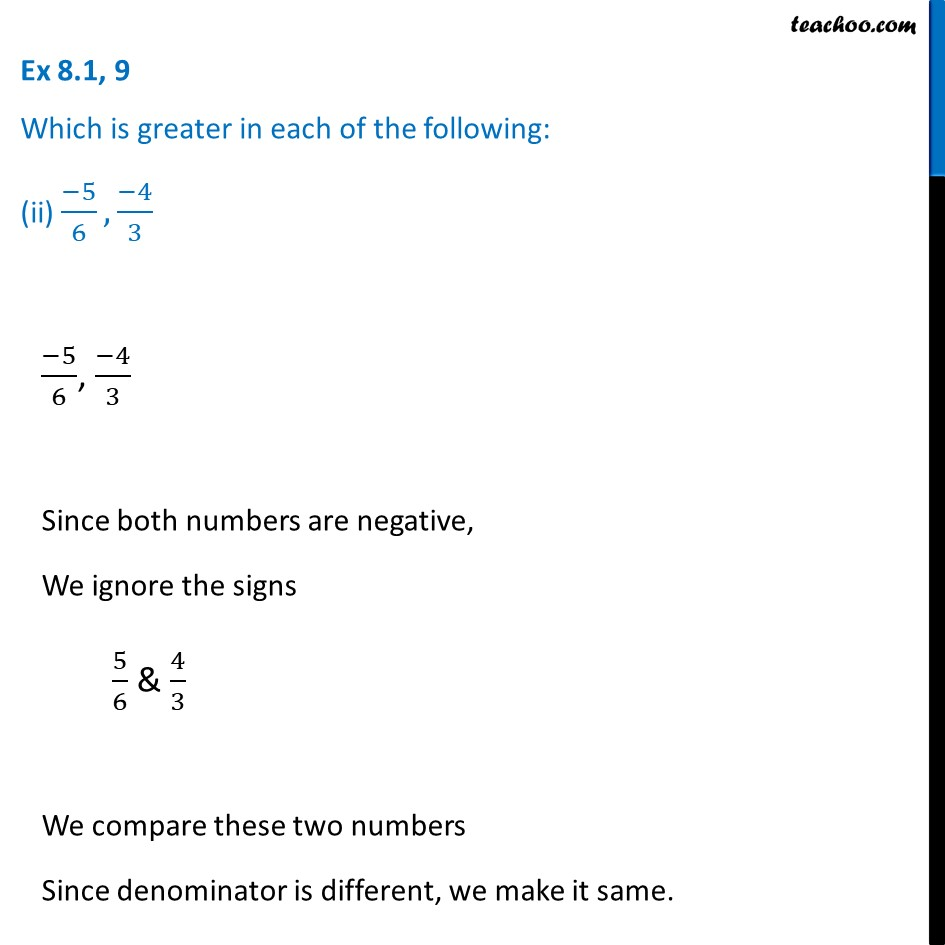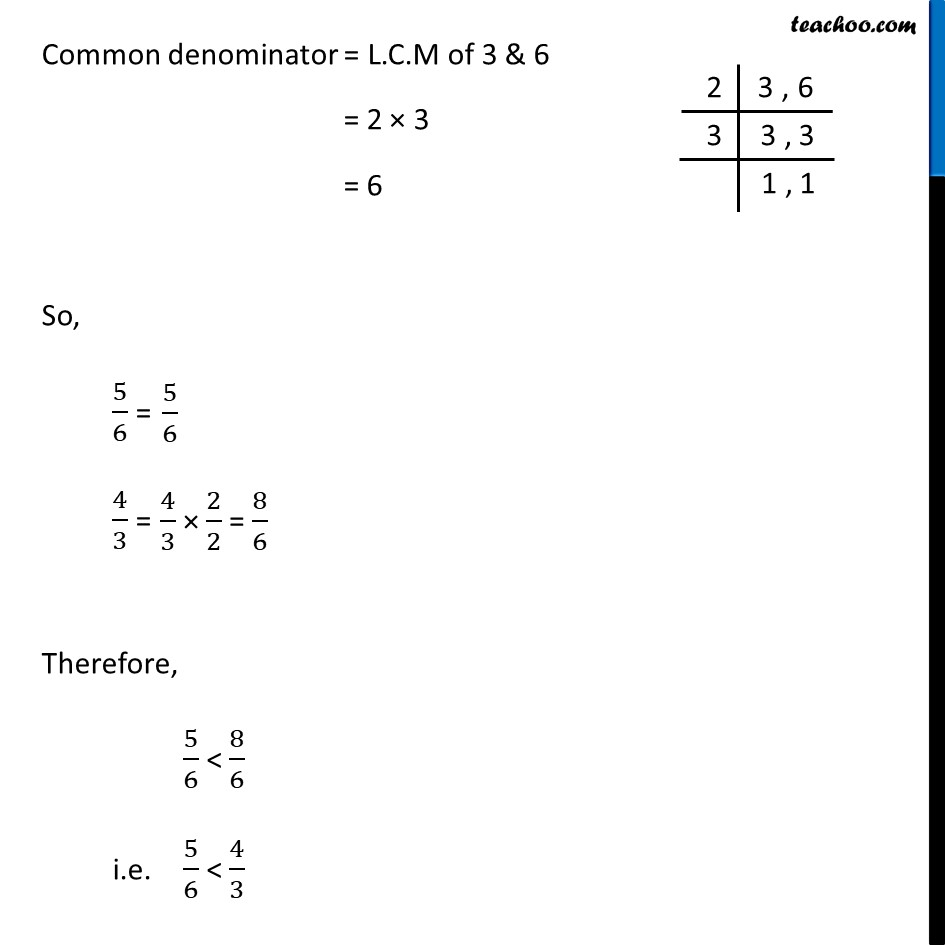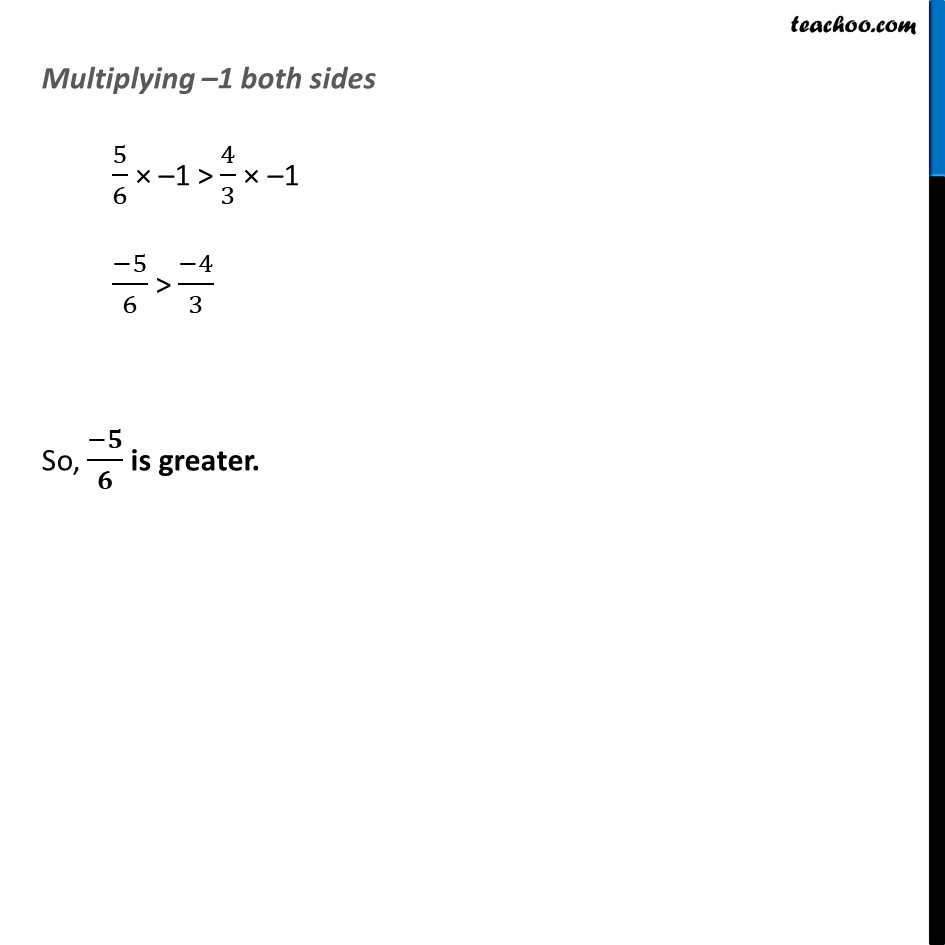Ex 8.1

Chapter 8 Class 7 Rational Numbers
Serial order wiseLearn in your speed, with individual attention - Teachoo Maths 1-on-1 Class

### Transcript

Ex 8.1, 9 Which is greater in each of the following: (ii) (−5)/6, (−4)/3 (−5)/6, (−4)/3 Since both numbers are negative, We ignore the signs 5/6 & 4/3 We compare these two numbers Since denominator is different, we make it same. Common denominator = L.C.M of 3 & 6 = 2 × 3 = 6 So, 5/6 = 4/3 = Therefore, 5/6 < 8/6 i.e. 5/6 < 4/3 Multiplying –1 both sides 5/6 × –1 > 4/3 × –1 (−5)/6 > (−4)/3 So, (−𝟓)/𝟔 is greater.